# 11 Scenarios

• What is a scenario.
• Create a scenario.

A scenario is a what-if model. It allows you to explore and calculate different future situations (scenarios) without changing values in the worksheet.

In a scenario, you give some variable cells (known as changing cells) different values and calculate the effect of the changes on certain result cells. The result cells contain formulas with references to the changing cells.

You might want to compare best-case and worst-case scenarios for the annual profit of a coffee shop, based on different costs. The result cell is the cell with the calculated profit. The changing cells are the cells with the costs.

Identifying the changing cells and result cells is key for creating scenarios.

With Excel’s scenario manager, you can create different sets of input data that calculate the corresponding results. You can save such a scenario under an appropriate name (such as Most Positive, Most Negative, …). You can easily compare the different results in a produced summary report. This report shows both the input values for the changing cells and the values of the result cells.

It is recommended to always save the current situation in a scenario, for example under the name Current.

## 11.1 Task: Coffee bar “The Brown Bean”

In figure 11.1, you see a calculation model of a coffee bar. The gray cells are the variable cells.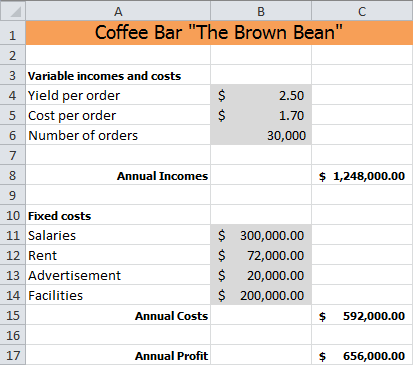Figure 11.1: Calculation model for coffee bar.

In addition to the current situation, the owner of the coffee bar wants to explore two other scenarios to see the effect on the profit.

Higher prices, less salary and advertising
A scenario with 10% higher prices and less investment in salaries ($30,000 less) and advertising ($ 5,000 less)
Higher prices, lower costs and more advertising
A scenario with 5% higher prices, 5% lower order costs and more advertising (€2000 more)

File: BrownBean.xlsx

1. Open the practice file.

Study the model. Identify the changing cells and the result cell. Study the used formulas and see that all cells have names.

If you give meaningful names to the cells then these names will be used in the summary report instead of the addresses. Then it will be easy to read the report.

1. Choose tab Data > What-If Analysis (group Forecast) > Scenario Manager.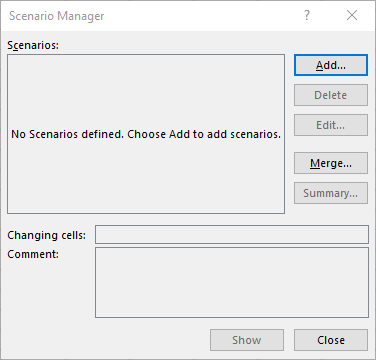Figure 11.2: Dialog box Scenario Manager.

2. Fill in the dialog box as shown in figure 11.3. When specifying the Changing cells, use the CTRL key to select multiple areas.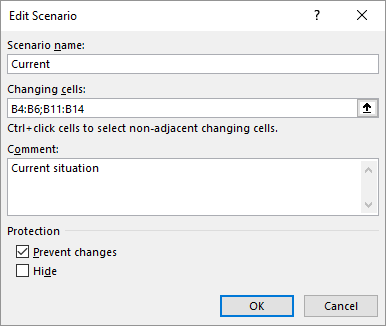Figure 11.3: Dialog box to record the current situation in a scenario.

1. Click OK. The window Scenario Values appears with the values for the changing cells already entered.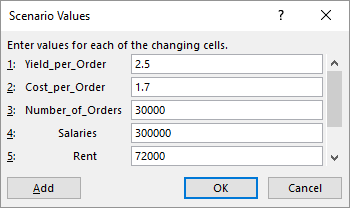Figure 11.4: Dialog box with the values of the changing cells for scenario Current.

1. Click on Add for entering a new scenario.

2. Give the scenario the name Higher prices, less salary and advertising. Give it a brief description and click OK.

The Scenario Values dialog box appears, still showing the current values of the changing cells.

1. Now type the new values in the cells that change for this scenario. Instead of calculating these values yourself, you can enter a formula. Excel automatically calculates the new value.

• Yield_per_Order: =1.1*2.5
• Salaries: =300000-30000
2. Click OK.

When you have used formulas, a warning screen appears that the entered formulas will be replaced by the results of the formulas.

Confirm with OK

1. The same way, create another scenario with the name Higher prices, less order costs and more advertising and adjust the values for this scenario.

After the last scenario has been added, you will return to the Scenario Manager dialog which now contains three scenarios (figure 11.5)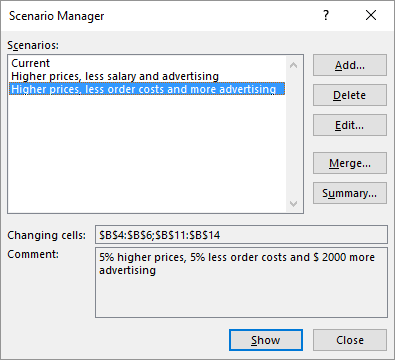Figure 11.5: Dialog box with the three scenarios.

1. Now select each scenario one after the other and then click on Show.

In the worksheet, the values in the changing cells and the cells that depend on them are adjusted for the selected scenario.

1. Click on Summary to create a report.

A dialog box will appear (figure @ref(fig:coffee bar-scene-reporttype)) in which you can choose the type of summary. Also the result cell (the cell with the profit) has already been filled in by Excel.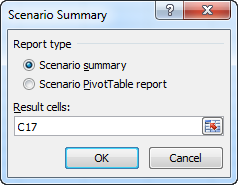Figure 11.6: Dialog box scenario summary.

1. Click OK.

A summary report will now appear in a new worksheet. Note that the left column contains the assigned cell names and not the addresses, making the report easier to read.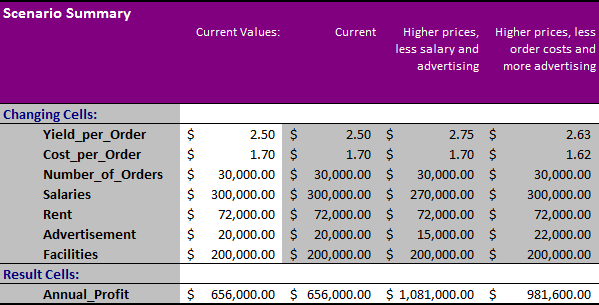Figure 11.7: Summary report.

## 11.2 Exercises

### scen001 - Profit margin - Sales price

Create the following table as accurate as possible. Use formulas for the determination of the margin, VAT, and the selling prices.Use the Scenario Manager to determine the selling prices (incl. VAT) for successively the profit margin rates of 15%, 20%, 25%, and 30%. The output should look like the following:File: Scen001.xlsx

### scen002 - Budget sports club

In the following figure, you see a simplified budget of a sports club. The rent is exactly known. For the other posts, a reasonable indication of the lower and upper limits can be given.

• The subscription revenues are between $1800 and$2400.
• The canteen revenues are between $600 and$800.
• The costs of materials are between $500 and$800.
• The miscellaneous costs are between $400 and$700.Enter the model in a worksheet. Create with the Scenario Manager the most positive and most negative scenario. The output should look like the following:File: Scen002.xlsx

### scen003 - Scenarios business profit

A company makes three products (A, B en C). To manufacture each product a different number of working hours and materials is needed. In the following figure, you see the rates for labor and material costs as well as the quantities needed to make these products. Also, the costs and revenues are calculated for the current production quantities.Enter the model in a worksheet. Provide the right formulas in the cells in which values have to be calculated.

Management wants a prediction of the operating profit, but there is still some uncertainty about the amount of labor and material costs. For the prediction are therefore three scenarios available: the favorable case, the bad case, and the current (most likely) case. The different rates are listed in the following table.

scenario wages material costs
favorable $60$ 114
bad $76$ 124
current $68$ 118

Create a summary report for these three scenarios using the Scenario Manager.

File: Scen003.xlsx### scen004 - Gainings automation company

The revenues and expenditures of an automation company for 2010 are listed in the following figure. The executive board would like to make a profit forecast for the years 2011 and 2012. The revenues and expenditures for these two years are influenced by the following factors.

• An increase of all revenues with a growth factor which is currently 10%.
• An increase of all expenditures except interest by an inflation factor which is currently 3%.
• The interest rate which is currently 8%.
• The tax percentage which is currently 35%.Create this model in a worksheet. The revenues and expenditures for 2010 are fixed and these should be calculated for 2011 and 2012. Provide the right formulas in the cells whose values have to be calculated.

Management wants a prediction of the profit forecast, but there is still some uncertainty about the development of some factors that affect the profit. The central planning bureau has released predictions of these factors. Based on these predictions three scenario’s are computed:Create a summary report for these three scenarios using the Scenario Manager.

File: Scen004.xlsx